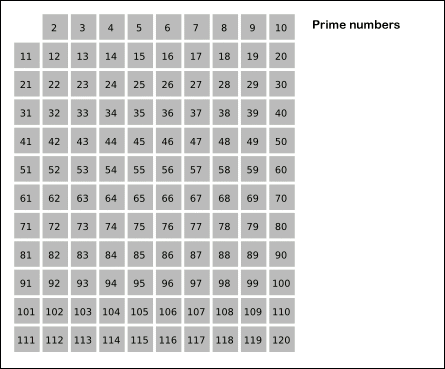# 204. Count Primes

Count the number of prime numbers less than a non-negative number,  n.

Example:

``````Input: 10
Output: 4
Explanation: There are 4 prime numbers less than 10, they are 2, 3, 5, 7.
``````

References:

How Many Primes Are There?

Sieve of Eratosthenes

Credits:
Special thanks to @mithmatt for adding this problem and creating all test cases.``````class Solution {
public:
int countPrimes(int n) {
int res = 0;
vector<bool> prime(n, true);
for (int i = 2; i < n; ++i) {
if (!prime[i]) continue;
++res;
for (int j = 2; i * j < n; ++j) {
prime[i * j] = false;
}
}
return res;
}
};
``````

Github 同步地址：

https://github.com/grandyang/leetcode/issues/204

Ugly Number II

Ugly Number

Perfect Squares

https://leetcode.com/problems/count-primes/

https://leetcode.com/problems/count-primes/discuss/57588/My-simple-Java-solution

LeetCode All in One 题目讲解汇总(持续更新中…)

 微信打赏Venmo 打赏×

Help us with donation